﻿ 基于朴素贝叶斯分类与群智感知实现对传染病与相应舆论的实时监控 Building Real-Time Monitoring of Infectious Diseases and Corresponding Public Opinion Based on Naive Bayesian Algorithm and Crowd Sensing

Operations Research and Fuzziology
Vol.08 No.03(2018), Article ID:26015,11 pages
10.12677/ORF.2018.83012

Building Real-Time Monitoring of Infectious Diseases and Corresponding Public Opinion Based on Naive Bayesian Algorithm and Crowd Sensing

Xuan Tang, Huajie Shen

Business School, Sichuan University, Chengdu Sichuan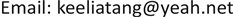Received: Jul. 3rd, 2018; accepted: Jul. 13th, 2018; published: Jul. 20th, 2018ABSTRACT

[Background/Meaning] The Weibo users are regarded as perceptual units to form a Crowd-sensing network, to produce intuitive and visual description of the distribution, transmission and development of infectious diseases and corresponding public emotions. [Method/Process] The study is divided into five parts: first, crawl the microblog posts associated with the event; second, use the data filtering model to filter the set of posts that have low correlation with the event; meanwhile, query and detect the position based on OpenStreetMap to return to the real position set; then, based on JIEBA word segmentation system of Python and Bayesian algorithm, achieve keyword emotional prediction; finally, through the emotion feedback model μ and epidemic monitoring model э, generate emotional curve and epidemic map, and make a case study. [Result/Conclusion] By this method (containing four models), epidemic emotion maps of infectious diseases and public emotion curves can be generated from Weibo. Among them, the map shows the epidemic situation and spread of each location set, and the emotion curve gives the public feedback changes. The study found that the more cases and the higher flow of population, the more negative the emotional performance. Excellent medical conditions and positive social media campaigns have a positive effect on public emotion.

Keywords:Crowd Sensing, Naive Bayes, Epidemic Monitoring, Emotional Recognition, Web Crawler[背景/意义]将微博用户看作感知单元，形成群智网络，从公众情感角度对传染病的分布、传播与发展进行简洁直观、可视化的描述。[方法/过程]研究分五个部分。首先，爬取与事件相关的微博帖集；其次，利用数据过滤模型α过滤与事件关联度不高的帖集；同时，基于OpenStreetMap查询并检测位置以返回真实位置集；然后，基于Python的JIEBA分词系统，进行分词和词频处理，并基于朴素贝叶斯算法实现对关键词的情感预测，得到情感集；最后，通过情感反馈模型μ与疫情监控模型э产生情感曲线与疫情地图，并进行案例研究。[结果/结论]通过这种方法(内含四个模型)，可以从微博上生成传染病事件的疫情情感地图与公众情感曲线。其中，地图展示了各位置集的疫情严重情况与传播情况，情感曲线给出了公众反馈随时间的变化。研究发现，病例数越多与人口流动性较大的地区情绪表现更为消极，优良的医疗条件和积极的社交媒体宣传对公众情绪有一定积极效果。1. 引言

2. 文献综述

2.1. 群智感知

2.2. 基于朴素贝叶斯的情感识别

2.3. 疫情监控地图

2.4. 基于社交媒体的疫情舆论

2.5. 评述

3. 框架概述

4. 模型概述

4.1. 数据过滤模型α

1) 变量定义

$Y\left({y}_{1},{y}_{2},\cdots ,{y}_{n}\right)$ 表示疫情事件集合， $S\left({s}_{\text{1}},{s}_{\text{2}},\cdots ,{s}_{n}\right)$ 表示相关搜索种子集， $RM\left(r{m}_{\text{1}},r{m}_{\text{2}},\cdots ,r{m}_{n}\right)$ 表示相关的帖子集， $E\left({e}_{1},{e}_{2},\cdots ,{e}_{n}\right)$ 表示采集数据集， $K\left({k}_{1},{k}_{2},\cdots ,{k}_{n}\right)$ 表示关键词集，  表示帖子时间集， $P\left({p}_{1},{p}_{2},\cdots ,{p}_{n}\right)$ 表示帖子位置集，zi，ai分别表示rmi帖子的转发量和获赞数。

2) 输入与输出

3) 规则定义

$\forall {E}_{i}\in E\to \left(\exists {s}_{j}\in S\wedge {s}_{j}\in r{m}_{j}\right)$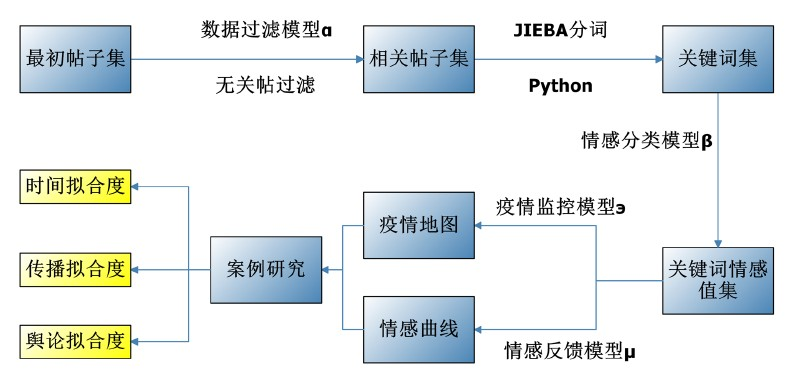Figure 1. Research framework

$\forall {E}_{i}\in E\to \left(\exists {t}_{y}\in T\wedge \exists {t}_{rm}\in T\wedge {t}_{rm}\in {t}_{y}\right)$

$\forall {E}_{i}\in E\to \left\{\exists {z}_{j}\in Z\wedge {a}_{j}\in A\wedge \left({z}_{j}\ge 1000\vee {a}_{j}\ge 1000\right)\right\}$

4) 模型过滤性能

4.2. 情感分类模型β

1) 变量定义

$D\left({d}_{1},{d}_{2},\cdots ,{d}_{n}\right)$ 表示分词集，  表示关键词di的情感值，M、H、L分别表示中性、积极、消极类情感， ${f}_{i}\left(1\le i\le n\right)$ 表示分词di的出现频率。ф表示各集合情感值。

2) 输入与输出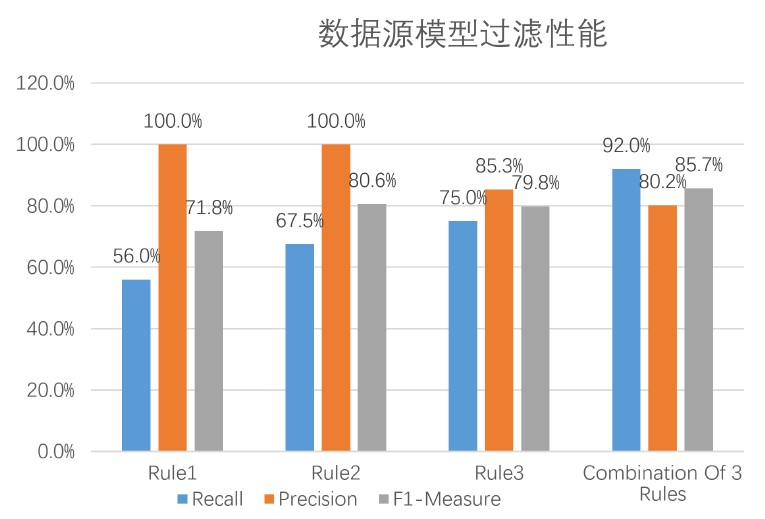Figure 2. Filtering quality

3) 规则定义

$\forall {\varphi }_{i}\in \varphi \to \left\{\exists {\text{k}}_{j}\in K\wedge {k}_{j}\in \left(L\vee H\vee M\right)\right\}$

$\forall {\varphi }_{i}\in \varphi \to \left\{{\varphi }_{j}={\sum }_{k=0}^{{n}_{j}}\left({\varphi }_{j}\ast {f}_{j}\right)/{n}_{j}\wedge {\varphi }_{j}\in \left[-1,1\right]\right\}$

4) 模型性能

4.3. 情感反馈模型μ

1) 变量定义

$MO\left(m{o}_{1},m{o}_{2},\cdots ,m{o}_{n}\right)$ 表示关键词月集合，ф表示月集合情感值，Ë表示情感值变化曲线。

2) 输入与输出

3) 规则定义

$\exists {e}_{i}\in \stackrel{¨}{E}\to \left\{\exists {e}_{j}\in \stackrel{¨}{E}\wedge unit=\left(MONT{H}^{\prime }\right)\right\}$

$\exists {e}_{i}\in \stackrel{¨}{E}\to \left\{\exists {\varphi }_{j}\in \varphi \wedge {\varphi }_{j}={\sum }_{k=0}^{{n}_{j}}\left(M{O}_{j}\ast {f}_{j}\right)/{n}_{j}\right\}$

4.4. 疫情监控模型э

1) 变量定义

$PO\left(p{o}_{1},p{o}_{2},\cdots ,p{o}_{n}\right)$ 表示关键词位置集合， $e{p}_{i}\left(1\le i\le n\right)$ 表示i位置的疫情值，MA表示疫情监控地图。

2) 输入与输出

3) 规则定义

$\forall m{a}_{i}\in MA\to \left\{\exists p{o}_{j}\in PO\wedge num\left(PO\right)=34\right\}$

$\forall m{a}_{i}\in MA\to \left\{\exists p{m}_{j}\in PM\wedge unit=\left(PROVINC{E}^{\prime }\right)\right\}$

$\forall m{a}_{i}\in MA\to \left\{\exists \in PM\wedge \exists e{p}_{j}\in EP\wedge e{p}_{j}=\left(p{m}_{j}\ast 2+1\right)\ast 255\right\}$

$\forall m{a}_{i}\in MA\to \left\{\exists {e}_{j}\in \stackrel{¨}{E}\wedge unit=\left(YEA{R}^{\prime }\right)\right\}$

5. 案例研究

1) 情感反馈模型结果

2017年1月~2017年3月，山东、玉林两例重症H7N9病毒感染患者被成功治愈，公众对于“H7N9”的情感反馈逐渐上升，由−0.248上升至−0.164，达到2017年的第一个极值点。

2017年3月~2017年6月，国家卫生计生委发布公告，多例患者已被治愈，H7N9的传播已得到控制，社交媒体用户的情感倾向持续上升，由−0.164上升至−0.047。

2017年6月~2017年7月，夏季是各类传染病的高发季，全国再次出现约10例确诊为H7N9病例，公众的情感值小幅下降至−0.066。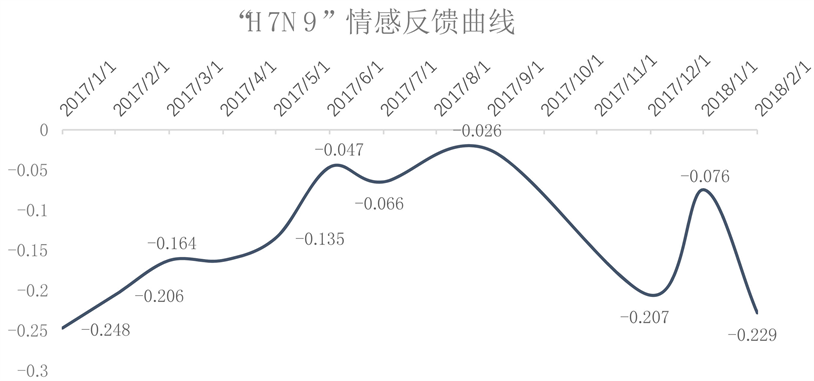Figure 3. “H7N9” event emotion curve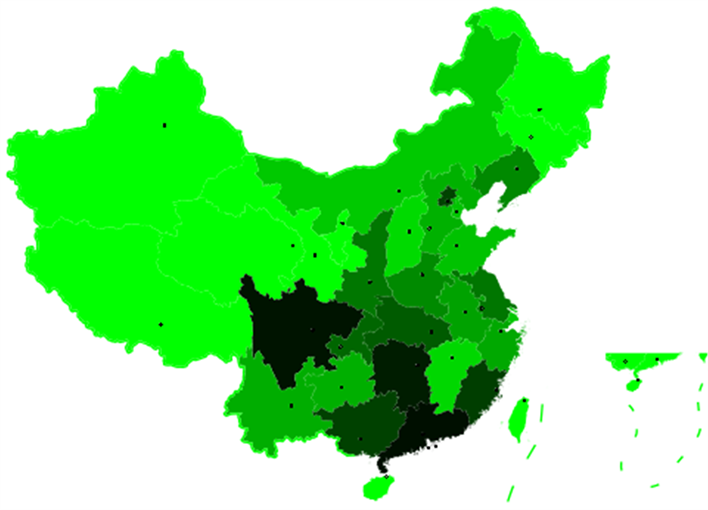Figure 4. Epidemic emotion map

2017年7月~2017年9月，复旦大学基础医学院应天雷课题组发现抗H7N9禽流感新型高活性全人源抗体，引发了微博的热烈讨论，群众情感值上升至−0.026，为2017年情感倾向的峰值。

2017年9月~2018年1月，国家卫生计生委发布《全国法定传染病疫情报告》，引发了微博用户的热烈讨论，公众情感值再次下降，至−0.207，后于2018年1月出现回升，至−0.076。

2017年1月~2018年2月，每年的12月至次年3月间,是人感染禽流感高发时段,此期间涉禽市场的阳性率高于水平，国内再次出现多例患者确诊为H7N9病例，社交用户的情感值持续走低。

2) 疫情监控模型结果Table 2. The number of cases corresponding to the emotional value of each location set (the position set of case number 0 has been omitted)Table 3. Emotional value-case number correlation coefficient

**在0.01水平(双侧)上显著相关。

6. 结论

Building Real-Time Monitoring of Infectious Diseases and Corresponding Public Opinion Based on Naive Bayesian Algorithm and Crowd Sensing[J]. 运筹与模糊学, 2018, 08(03): 90-100. https://doi.org/10.12677/ORF.2018.83012

1. 1. Hong, L., Gurumurthy, S., Gurumurthy, S., et al. (2012) Discovering Geographical Topics in the Twitter Stream. Proceedings of the 21st International Conference on World Wide Web, Lyon, 16-20 April 2012, 769-778. https://doi.org/10.1145/2187836.2187940

2. 2. Lehmann, J., Gonçalves, B., Ramasco, J.J., et al. (2011) Dynamical Classes of Col-lective Attention in Twitter. 21st ACM International Conference on World Wide Web (WWW), New York, 251-260.

3. 3. Sakaki, T., Okazaki, M. and Matsuo, Y. (2010) Earthquake Shakes Twitter Users:Real-Time Event Detection by Social Sensors. Proceedings of the 19th international conference on World Wide Web, Raleigh, 26-30 April 2010, 851-860. https://doi.org/10.1145/1772690.1772777

4. 4. 赵东, 马华东. 群智感知网络的发展及挑战[J]. 信息通信技术, 2014(5): 66-70.

5. 5. Guo, B., Chen, H., Yu, Z., et al. (2015) FlierMeet: A Mobile Crowdsensing System for Cross-Space Public Information Reposting, Tagging, and Sharing. IEEE Transactions on Mobile Computing, 14, 2020-2033. https://doi.org/10.1109/TMC.2014.2385097

6. 6. Huang, J., Kornfield, R., Szczypka, G. and Emery, S.L. (2014) A Cross-Sectional Examination of Marketing of Electronic Cigarettes on Twitter. Tobacco Control, 23, iii26-iii30. https://doi.org/10.1136/tobaccocontrol-2014-051551

7. 7. 赵东. 移动群智感知网络中数据收集与激励机制研究[D]: [博士学位论文]. 北京: 北京邮电大学, 2014.

8. 8. Chen, Y.Y., Cheng, A.J. and Hsu, W.H. (2013) Travel Recommendation by Mining People Attributes and Travel Group Types from Community-Contributed Photos. IEEE Transactions on Multimedia, 15, 1283-1295. https://doi.org/10.1109/TMM.2013.2265077

9. 9. Pankratius, V., Lind, F., Coster, A., et al. (2014) Mobile Crowd Sensing in Space Weather Monitoring: The Mahali Project. IEEE Communications Magazine, 52, 22-28. https://doi.org/10.1109/MCOM.2014.6871665

10. 10. Lima, A.C. and De Castro, L. (2013) Multi-Label Semi-Supervised Classification Applied to Personality Prediction in Tweets. 2013 BRICS Congress on Computational Intelligence and 11th Brazilian Congress on Computational Intelligence, Ipojuca, 8-11 September 2013, 195-203. https://doi.org/10.1109/BRICS-CCI-CBIC.2013.41

11. 11. 彭蔚喆. 面向中文微博文本的情感识别与分类技术研究[D]: [硕士学位论文]. 武汉: 华中师范大学, 2014.

12. 12. 张扬, 崔晨阳. 基于朴素贝叶斯模型的一种网络负面信息预警策略研究[J]. 图书馆杂志, 2014, 33(8): 78-82.

13. 13. Ji, X., Chun, S.A., Wei, Z. and Geller, J. (2015) Twitter Sentiment Classification for Measuring Public Health Concerns. Social Network Analysis and Mining, 5, 1-25. https://doi.org/10.1007/s13278-015-0253-5

14. 14. Rosen, S., Lee, S.J., Lee, J., et al. (2014) MCNet: Crowdsourcing Wireless Performance Measurements through the Eyes of Mobile Devices. IEEE Communications Magazine, 52, 86-91. https://doi.org/10.1109/MCOM.2014.6917407

15. 15. 吴文乐, 郭斌, 於志文. 基于群智感知的城市噪声检测与时空规律分析[J]. 计算机辅助设计与图形学学报, 2014, 26(4): 638-643.

16. 16. 葛小三, 付魁, 程钢, 马勇, 孙玉祥. 数据挖掘支持下的网络热点事件地理可视化研究[J]. 河南理工大学学报(自然科学版), 2016, 35(5): 655-659.

17. 17. Broniatowski, D.A., Paul, M.J. and Dredze, M. (2013) National and Local Influenza Surveillance through Twitter: An Analysis of the 2012-2013 Influenza Epidemic. PLoS ONE, 8, e83672. https://doi.org/10.1371/journal.pone.0083672

18. 18. Kim, J. and Hastak, M. (2018) Social Network Analysis: Characteristics of Online Social Networks after a Disaster. International Journal of Information Management, 38, 86-96. https://doi.org/10.1016/j.ijinfomgt.2017.08.003

19. 19. 梁芷铭, 周玫, 宁朝波. 基于情感本体的网络舆情观点挖掘模型构建——政务微博话语权研究系列之十一[J]. 情报杂志, 2014, 33(5): 143-147.